Classical gravity is not good enough.  As a first step, one must think about groups since that is the foundation of the Standard Model of physics.

All my failed efforts to think about gravity in new ways were variations on the Maxwell equations.  Groups struck me as too hard.  I might never be able to comprehend SU(5), SU(10), or E8, where studies on unified fields take place.

There are a few things I know about the standard model.  The strong force is based on the gauge symmetry of the continuous Lie group SU(3).  There are eight generators in its Lie algebra, su(3), and so we know there are eight gluons.  Each of those generators has a norm of one.

The weak force has a gauge symmetry of SU(2), the unit quaternions.  The unit quaternions have a norm of one.  EM has the simplest gauge symmetry, U(1), complex numbers with a norm of one.  There is a pattern here involving a central role for unity.  Gravity is darn similar to EM, but somewhat simpler since there is only one sign for its charge instead of two signs for the charge of EM.  The only thing simpler than complex numbers with a norm of one would be the real numbers with a norm of one (Z2).

This pattern suggest a scalar model might work for gravity.

You have a measure of unity which references yourself, and it is one.  I have a measure of unity that references myself, and it is one.  If a third party compares our two measures in flat spacetime, the result is they are the same.  In curved spacetime, our measures of unity remain one.  But the comparison will reveal differences: your seconds get larger than mine but your meter stick gets smaller as you approach any gravitational source.  Unity comparisons can get complicated.

## Failures of All Scalar Gravity Models

Scalar models for gravity were the first ones developed, going back to the first giant in physics, Sir Isaac Newton.  Once Albert Einstein developed special relativity, it was obvious the gravity law needed to be changed.  Many things were tried (resources at the end).  One class of work assumed space-time was flat.  A fix would involve adding more terms just like EM.  That was a reasonable suggestion in the early 1900's, but we have far too much data saying space-time is curved via tests of the equivalence principle to take such proposals seriously.

One of the best scalar models was developed by Einstein and Fokker.  It at least worked in curved space-time (it was covariant).  Yet it suffered from a technical issue because it was not diffeomorphism invariant.  Even without that issue, the work suffered the same flaw as all other scalar gravity models:

All scalar gravity models predict there is no bending of light around the Sun, yet experiments have shown that light does bend, ergo all scalar gravity theories are wrong.

Examine this assertion by doing the work in detail.

## Calculating Light Bending

Let us practice the bending of light calculation on a model that works: general relativity.  There is the Schwarzschild solution to the GR field equations for a spherically symmetric, non-rotating, uncharged source.  The solution comes in the form of a metric, here written in isotropic coordinates: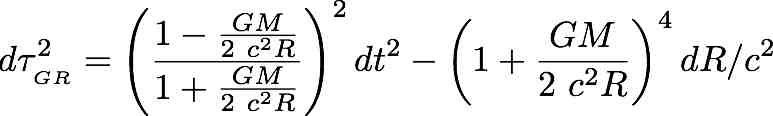For light, the interval will be zero.  One can solve for a velocity due to gravity, taking the Taylor series approximation due to the small size of the dimensionless gravity term even for the Sun: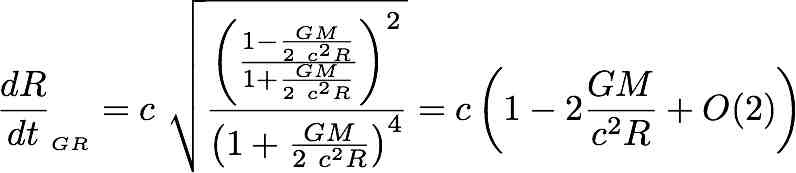One can interpret this gravitationally-induced slowdown in a non-inertial reference frame as acting like an index of refraction.  Consider a photon flying by the Sun, setting z=0: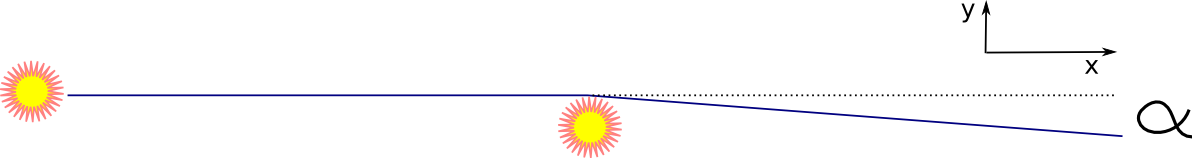The total transverse deflection will be the integral over all of x of the derivative of the velocity with respect to y.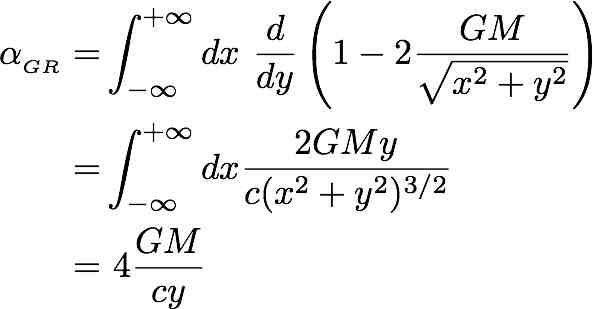There remains a historical debate about how good the initial data was for light bending during an eclipse.  Work done by researchers at the Haystack Observatory a few miles from my home using radio astronomy has confirmed that gravity bends light precisely this amount to first order.  A measure to second order is currently beyond our reach.

Scalar gravity models can be written as actions.  Here is one from Misner, Thorne, and Wheeler, problem 7.1.  Use a worldline z parameterized by lambda: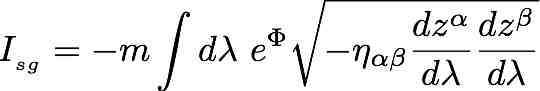[technical note: the m above is the inertial mass of a test particle which does not have to be a photon. To analyze photons, one needs to look at the limit as m goes to zero. This equation is for the vacuum because a matter term was not included in this initial analysis.]

[More important technical note: The literature on this topic invariably writes the interaction term with this m (MTW, Thirring). I followed that tradition.  In the discussion below, particularly by LagrangiansForBreakfast, it was pointed out that this m serves as a coupling constant, like the fundamental electric charge e does in EM.  There is no fundamental mass charge m.  In our best approach to gravity to date, general relativity, is pure geometry.  This m means the proposal is not pure geometry and it is not consistent with the equivalence principle.  This blog challenged a class of models in the literature, but was not quite bold enough.  I am working on a way to address the issue, but it will be in a separate blog.]

Notice the simplicity of the action since it is not a function of lambda or z, only the derivatives z'.  Vary the action with respect to the parameter lambda and look for an extremum value: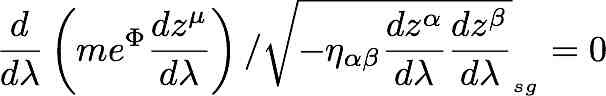Because the derivative is the constant zero, the term inside the derivative is a constant of motion.  What is this constant of motion 4-vector?  It is the 4-momentum: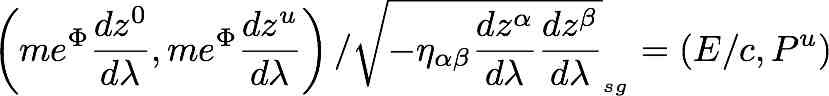For a photon, these two values will be equal.  Solve for the velocity.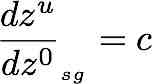There will be no bending of light for this scalar gravity model.  Even if one tries out exotic potentials, the same result happens every time.

Scalar gravity models

cannot

bend

light.

## Interlude: The Tense Marriage of Space and Time

In 1908, Minkowski predicted a bright future for the newly married space-time couple:

The views of space and time which I wish to lay before you have sprung from the soil of experimental physics, and therein lies their strength. They are radical. Henceforth space by itself, and time by itself, are doomed to fade away into mere shadows, and only a kind of union of the two will preserve an independent reality.

Always staying rooted in the soil of experimental physics is a good credo.  Yet the radical idea has not come to pass.  People worry about the "Arrow of Time".  It is a riddle destine to keep riddling because space has been cut out of the problem description.  Others think time is not fundamental, being emergent instead of space's equal partner.

The marriage of space and time is tense.  They have different units.  A factor of c is required to compare the two (you choose who gets the c).  In flat space-time, one can contract a space-time vector and get a number that all inertial observers agree upon.  That calculation grants positive values to one partner and negatives to the other (you choose who bears the minus signs).  The difference in signs has major consequences to the outcome of special relativity.

What happens to space-time due to gravity was unexpected.  In the contraction of a space-time vector, the previous constant values of plus and minus one were changed to dynamic values.  The dynamic value was the ratio of gravitational source mass to distance.  Just as important, what happens to measurements in space is approximately the inverse of what happens to measurements in time.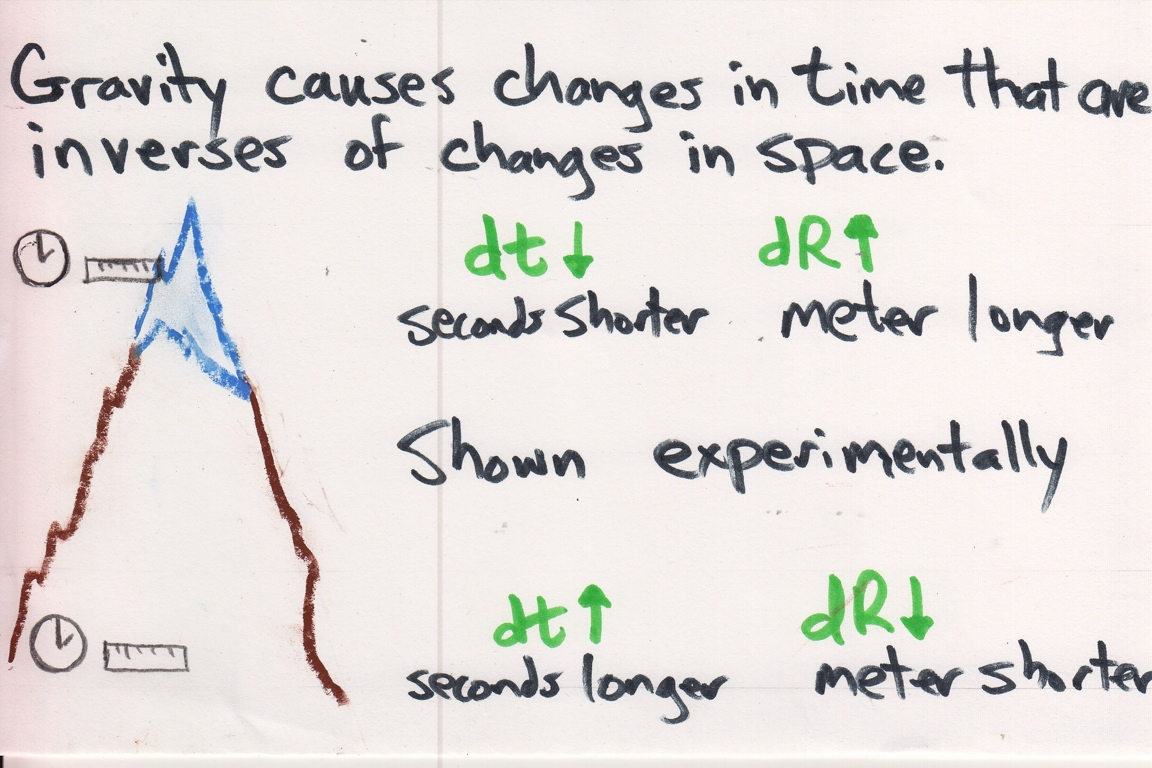Gravity makes seconds bigger (think black holes) while shortening meter sticks (everyone is getting closer).  This pattern is grounded in experimental physics.

## Scalar Gravity Coupling to the Worldline Correctly

The scalar gravity proposal everyone rightly dismisses has a contraction of a 4-vector that is re-scaled by a gravity term.  In other words, both time and space intervals either go up and down together as one moves around in space-time.  Rewrite the action with no Greek letters.  Bring in the gravity term next to the worldline: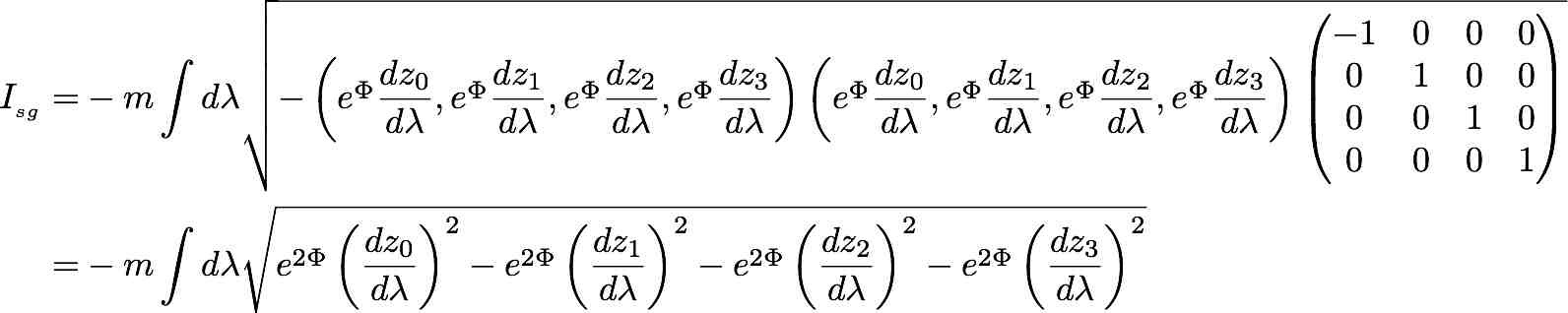Vary the parameter lambda when there is no gravitational mass.  The result is consistent with experiment.  Repeat when there is a weak gravitational field, and the scalar gravity model suggests that any changes in time - up or down - is matched in size and direction by a change in space.  Experiments have shown that to be false, so the action is not worthy of further consideration.

I propose the scalar gravity coupling (sgc) model.  Gravity couples to the worldline of the particle in a manner consistent with experiment.  The scalar gravity coupling action is: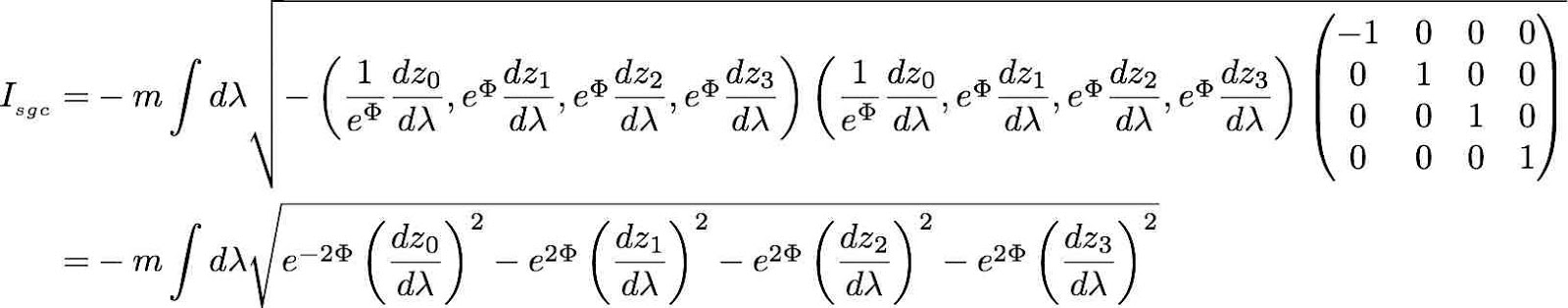[technical note: the m is the inertial mass of a test particle. At an appropriate time, for a photon the limit as m goes to zero will be defined. This action is for the vacuum since there is no matter Lagrangian included.]

The same scalar gravity term was used in this action as before.  In this model one cannot pull the scalar outside the square root.  This is neither about gravity nor the worldline, but instead about the relationship between the two.  When one varies the parameter lambda when there is no gravity, then the results are consistent with experiments in flat space-time.  When there is a weak gravity field, a variation that causes the time term to get larger will cause the space terms to shrinks and visa versa.  That behavior has a chance to be consistent with tests of weak field gravity.

The equations of motion are: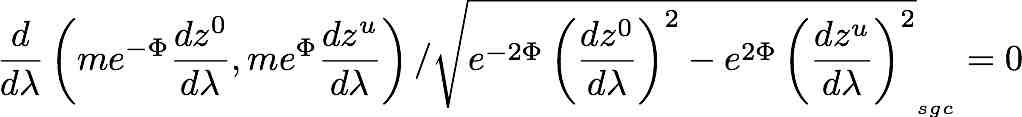[technical note: this is a force equation. It would apply to a geodesic if the parameter was the proper time of the test particle/]

The 4-momentum is a conserved quantity: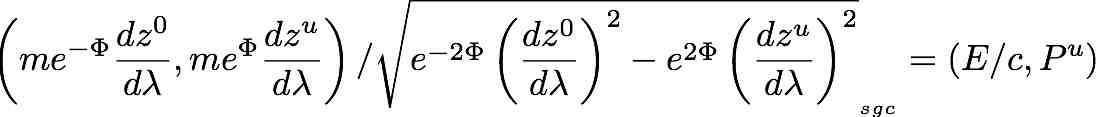[technical note: the analysis so far has not depended on the test particle being a massless photon. That limit is now investigated.]

For light, these two terms will be equal. This also allows one to cancel the test mass m. Calculate the deflection velocity: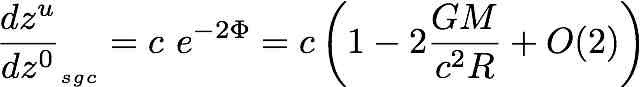To first order, this is the same deflection velocity as GR, so will have the same bending.

## Experimental Tests: GR versus sgc

The scalar gravity coupling model is not a metric theory.  It depends on only one dynamic variable which is insufficient to represent a metric that can require up to ten variables.  The model can be represented as a metric using this map: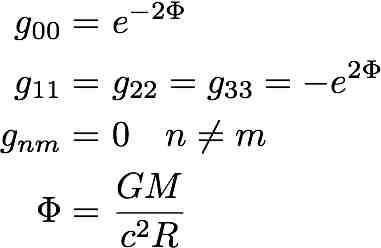This is known as the Rosen metric in the literature, which arises from a bi-metric model, one with a fixed background metric as well as a dynamic one.  The bi-metric model is an exact solution of the field equations of GR, while the exponential metric by itself is not.  The Rosen metric does curve space-time.  Rosen's model fails strong gravity field tests because it predicts dipole gravity waves.  Observations of gravitational energy loss by binary pulsars have shown that a quadrapole is the lowest mode of emission.  This strong field requirement is one of the hardest to satisfy, but the scalar gravity coupling model may do so since only one function and its inverse come into play.

How will the exponential metric representation do against general relativity for weak fields?  One needs to know the Parameterized Post Newtonian (PPN) form of the Schwarzschild solution. It uses the first three Taylor series terms of g00 and the first two terms of gRR: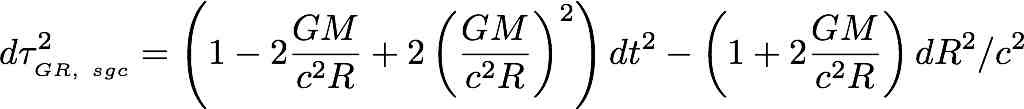These 5 terms are exactly the same five terms of the scalar gravity coupling model.  There is no weak gravity field test one could do to first order PPN accuracy to tell the difference between these two models.

At second order PPN accuracy, the story is different for light bending.  The third spatial term comes into play: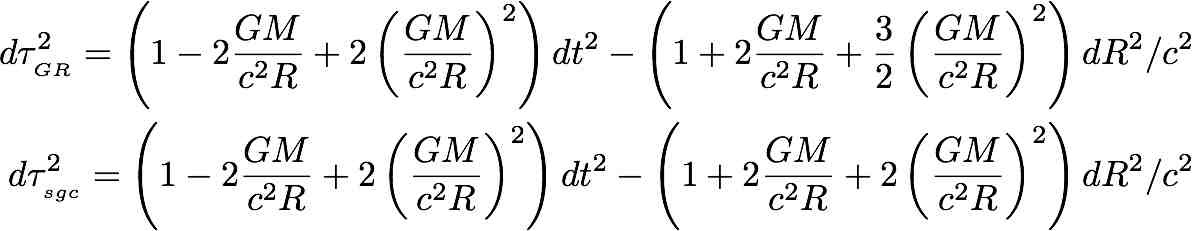Epstein and Shapiro provide a formula for the post-post Newtonian deflection (Phys. Rev. D, 22:12, p. 2947, 1980):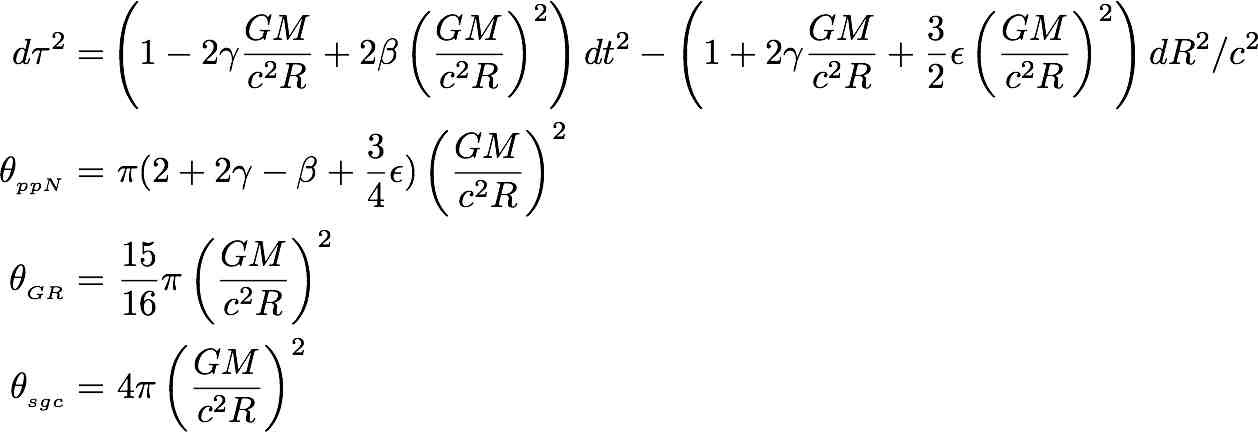For light bending around the Sun, this translates into a prediction of 11.0 microarcseconds for GR, and 11.7 microarcseconds for scalar gravity coupling.

[technical note: one needs to plug in Newton's gravitational constant G, the mass of the Sun M, the speed of light c, the radius of the Sun, then convert that value in radians to arcseconds to get the above values. I will leave that as an exercise for the reader.]

Notice that beta and eta terms cancel for the sgc model instead of leaving a -¼ factor behind as happens with GR.  A numerologist might be happy for the gravity coupling model that there is both more bending predicted, and the results are "neater".  Yet to remain grounded in the soil of experimental physics, only the data will matter.  We can measure light bending around the Sun to 100 microacrseconds.  Both the quadrupole moment of the Sun, and the fact that the Sun rotates could add as much as a microarcsecond to the light bending. It is good that the model can be accepted or rejected on experimental grounds, even if only in theory at this moment.  The scalar gravity coupling model predicts more bending than our best model and the measurement requires a technical feat beyond our means today (sound familiar?).

## Big Picture View

Newton's law of gravity remained unchallenged from its birth until 1905.  The advent of special relativity drew renewed research into alternative scalar gravity theories over the next 10 years.  The variation of the action techniques were used even though the physicists did not know how gravity changed the path due to the gRR term.  All models used would later be shown to be in conflict with experiment.  The triumph of general relativity in 1915 squelched nearly all but a few efforts to investigate scalar gravity theories.

The word "scalar" is used in two ways in this discussion.  First, a scalar field means it depends on one dynamic value for the field.  Second, a scalar value transforms like a tensor means that it behaves like any typical number.  In earlier proposals, the field depended on one value and transformed like a scalar in a tensor expression.  As such, it would increase values in time and space measurements.  In this coupling proposal, the function for the field depends on one value.  Yet by coupling that one function to space and its inverse to time, the variation principle applied to a world line is consistent with what gravity does to space-time.

Some will surely complain that this coupling trick is novel nonsense.  Tensor algebra does not allow one to write such an action. There is no other fundamental force that acts this way.  Tensor notation, powerful as it is, may have blinded others to seeing this possibility which is based on experimental data, not on math rules. Data should trump conventions.  There only has to be one force that does the work of gravity, which is to curve space-time.  We do not have to fight about the proposal.  Much better would be to fund the measurement of light bending and let the data decide.

The introduction used an appeal to group theory as a motivator for this investigation.  All the work that followed was completely classical.  What scalar gravity coupling does is change the length of any and all 4-vectors and higher ordered tensors, no matter what goes into said tensors.  If such tensors are used in a calculation of a quantum effect, both the probability amplitude and probabilities will change, but not the form of the calculation.  If that turns out to be the case, there might not be a need for the graviton.  Instead, particles like light represent the curvature of space-time via their coupling to paths in space-time.  The graviton would join the ether in the crushing minimalism of physics.  Or not :-)

#### Resources:

"Gravitation" by Misner, Thorne, and Wheeler, problem 7.1 in particular.

# "Einstein Einstein and Nordstrom: Some Lesser-Known Thought Experiments in Gravitation" in "The Attraction of Gravitation: New Studies in the History of General Relativity"

edited by John Earman, Michel Janssen, John D. Norton

### Gravitational Bending of Light, Don Edwards

http://home.fnal.gov/~syphers/Education/Notes/lightbend.pdf, 2007

Wiki pages
Alternatives to General Relativity

Scalar theories of gravitation

Diffeomorphisms